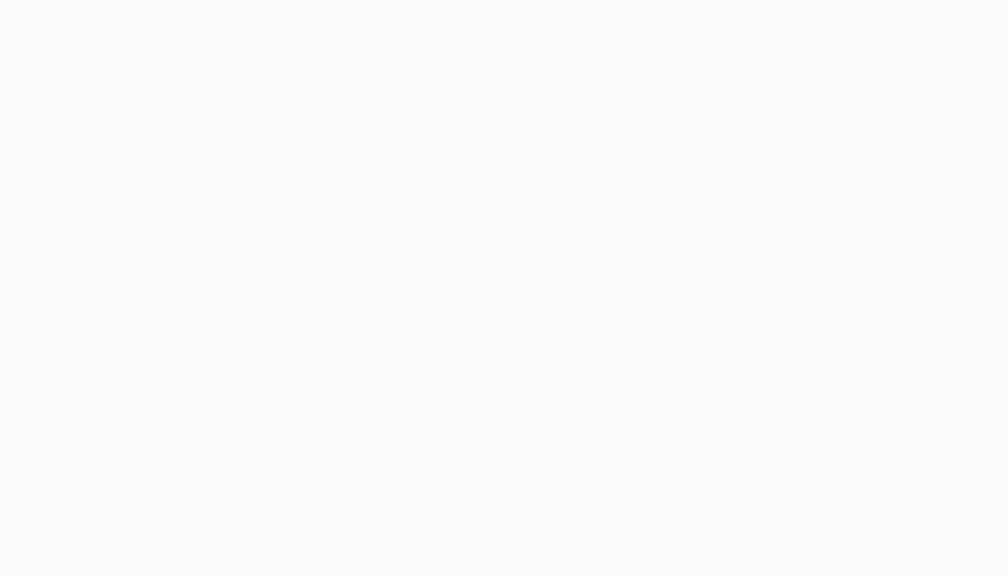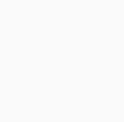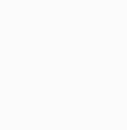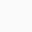# BECE Mathematics Trial Test for JH3 students (Test 2)This is another BECE Mathematics Trial Test for JH3 students (Test 2) aimed at helping learners and teachers to revise what was learnt in previous classes at the Junior High School.

All students who attempt these objective and subjective questions are encouraged to show working and also show their final answers to their Mathematics teachers.

These questions are also excellent for revision and Mathematics teachers can make good use of these for JHS3 and other levels to re-enforce the lessons taught.

## BECE Mathematics Trial Test for JH3 students (Test 2)

1. Simplify 2b+a+4b
A -6a+2b B. 6b +a C. -2b + a D. 6a + b

2. What is the place value of the underlined digit 7418920

A. hundred B. thousand C. million D. ten thousand?

3. A set which has one element is called

A. unit set B. infinite set C. empty set D. disjoint set

4. The distance from a centre of a circle to any point of the circumference is called

A. radius B. sector C. diameter D. segment

5. How many faces have a cone
A. 2 B. 3 C. 4 D. 5

6. Which of the following is not a prime number?

A. 1 B. 2 C. 23 D. 5

7. State the property used in the operation a(b + c) =ab +ac

A. associative B. commutative C. Distributive D. Universal

8. How many vertices has a cuboid A. 6 B. 7 C. 8 D. 14

9. Find the median of the number 7, 2, 5, 6, 8, 8, and 13
A. 7 B. 6 C. 8 D. 5

10. A boy scored 17/25 in a mathematics text. Express his score as a percentage
A. 17% B. 68% C. 85% D. 65%

11. What is the perimeter of a rectangle with length 7cm and with 5cm
A. 35cm B. 24cm C. 19cm D. 17cm

12. What is the name of the figure below?A. equilateral triangle B. rectangle triangle C. scalene triangle D. isosceles triangle
13. Change 0.32 to fraction
A. 32/1000 B. 32/100
C. 32/10 D. 3.2/100
14. The most frequently occurring value in a set of data is called………… A. mode B. median C. mean D. average
15. Convert 25% as common fraction
A. 1/4B. 3/20
C. 2/10D. 2/4
A. 8230
B. 8130
C. 7241
D. 3521

17. Find the area of the figure belowA. 27cm2
B. 21cm2 C. 28cm2
D. 26cm2

18. Which of the following numbers have only two factors    A. 9 B. 31 C. 15 D. 22

19. Round 724 to the nearest hundred     A. 700 B. 800 C. 720 D. 730

20. Simplify 1013÷ 107       A. 103B. 106C. 1020D. 1017

21. Write the number name for 5,234
A. five thousand two hundred and thirty-four
B. fifty two thousand, two hundred and three
C. five thousand, two hundred and four
D. five million, two hundred and thirty-four

22. What is the HCF of 24 and 40?      A. 10 B. 6 C.8 D. 12

23. Simplify 14pq × q    A. 14pq2  B. 14p2q C. 14pq D. 14p2q2

24. The distance from one point on the circle to another point on the circle without passing through the Centre is called       A. radius B. chord C. Diameter D. Sector

25. Which of the following is an infinite set?
A. {5, 7…1001}
B. {5, 9, 15, 19}
C. {17, 19, 21, 23…}
D. {2, 4…62,64,…122}

26. write five hundred and five million, five hundred and five in figures A. 55550000 B. 5055050000 C. 505000505 D. 505005005

27. Simplify 322×43 A. 218 B. 216 C. 210 D. 26

28. Which of the following is arranged in descending order?
A. -32, -2, 6, 21 B. -2, -32, 6, 21 C. 21,6, -32, -2 D. 21,6,-2,-32.

29. (x )/4=2     A. 2 B. 4 C. 6 D. 8

30. Find the product of 17 and 121.  A. 968 B. 1751 C. 2057 D. 8,591
31. Express 2/5 as a percentage A. 20% B. 25% C. 40% D. 80%

Use the information below to answer questions 32 and 33

The following are the marks obtained by pupils in a test; 2,3,5,2,3,4,2,3,5,3

32. What is the mode? A. 2 B. 3 C. 4 D. 5

33. Calculate the mean A. 3.0 B. 3.2 C. 4.0 D. 4.2

34. Find the set of the integers within the interval -2<x<2 A. {2,1,2} B. {2,1,0} C. {-1,0,1} D. {1,1,2}

35. Which of the following statements is true? A. 8+4<10B. 7+4<10 C. 6+4<10 D. 5+4<10

36. What is the name of the polygon below? A. Pentagon B. octagon C. hexagon D. quadrilateral

37. Which of the following is the set of prime factors of 12?
A. {1,3} B. {2,3} C. {2,4,6,12} D. {2,3,4,6}

38. The value of an obtuse angle lies between
A. 0° and 90° B. 90° and 180° C. 90° and 270° D. 180° and 360°

39. How many days are in a year?
A. 365 B. 366 C. 356 D. 400
40. Expand the expression 2(3a+2b
A. 6a + 2b B. 5a +4b C. 6a +4b D. 10ab

## SECTION BAnswer any three (3) questions

Q1

a. Express the following decimals as fractions in their simplest form i) 0.45
ii)0.75

iii) 0.8

Q2

If X={natural numbers up to 15}.
Form these sets from set X
ii) M={even numbers between 1 and 10}
iii) N={prime numbers less than 10}
iv) M∩N.

A die is thrown 20 times with the following results
6,1,6,5,4,3,3,6,1,6,
4,1,2,5,6,5,3,6,2,1
a) Make a frequency table for the above results
(b) Find
i)The mean
ii) The median
iii) The mode from the table

Q4.
Convert the following fractions to percentages

ii) 12/20

iii) 8/10
Simplify
a) 14pq × q
b) 2(3a+2b)
c) 322×43

We hope these questions titled BECE Mathematics Trial Test for JH3 students (Test 2) would be useful to our cherished parents, teachers and students

## One thought on “BECE Mathematics Trial Test for JH3 students (Test 2)”

1.Boateng Godfred says:

Am interested